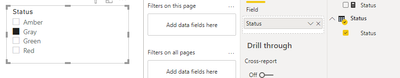cancel
Showing results for
Did you mean:Helper I

## How to use a measure as slicer?

Hi, I have a measure which categorise my data based on few statuses. How can I use this status measure as a slicer?

Status = IF(ISBLANK(Difference),"Gray", IF(Difference>0, "Green", IF(Difference<0, "Red", "Amber")))

- Difference is a measure as well-

I need a slicer with Gray, Green, Red , Amber so users can filter the data base on this. I have to use a measure for the status as a calulated column will not give me the desired results. I cant drag the measure as a slicer.
Thanks.

1 ACCEPTED SOLUTIONCommunity Support

Hi @yybi123 ,

Based on your description, you can so some steps as follows.

Here is my test table.I create two measures as follows.

`Difference = MAXX('Measure_Slicer_test',[D2]-[D1])`
`Status = IF(ISBLANK([Difference]),"Gray", IF([Difference]>0, "Green", IF([Difference]<0, "Red", "Amber")))`
1. Create a calculated table.
`Status = var x1=SUMMARIZE('Measure_Slicer_test',Measure_Slicer_test[Product],"Status",[Status])returnSUMMARIZE(x1,[Status])`2. use "Status"[Status] to create a slicer.3. create a measure, then drag it to the table visual of the original table and set the value as "1".

`Measure_filter = var x1=SELECTEDVALUE('Status'[Status])returnIF(ISBLANK(x1),1,IF([Status]=x1,1,0))`Result:Hope that's what you were looking for.

Best Regards,

Yuna

If this post helps, then please consider Accept it as the solution to help the other members find it more quickly.

7 REPLIES 7New MemberAnonymous
Not applicable

Hi,
I want to use measure in the slicer. And that measure consist of another two measures. So, I am not able to acheive this.Community Support

Hi @yybi123 ,

Based on your description, you can so some steps as follows.

Here is my test table.I create two measures as follows.

`Difference = MAXX('Measure_Slicer_test',[D2]-[D1])`
`Status = IF(ISBLANK([Difference]),"Gray", IF([Difference]>0, "Green", IF([Difference]<0, "Red", "Amber")))`
1. Create a calculated table.
`Status = var x1=SUMMARIZE('Measure_Slicer_test',Measure_Slicer_test[Product],"Status",[Status])returnSUMMARIZE(x1,[Status])`2. use "Status"[Status] to create a slicer.3. create a measure, then drag it to the table visual of the original table and set the value as "1".

`Measure_filter = var x1=SELECTEDVALUE('Status'[Status])returnIF(ISBLANK(x1),1,IF([Status]=x1,1,0))`Result:Hope that's what you were looking for.

Best Regards,

Yuna

If this post helps, then please consider Accept it as the solution to help the other members find it more quickly.Frequent Visitor

Great solution representing the inguinity of using the OOB PBI capablities.

Thank you !New Member

Hi Team,

If One date is fixed and another date is selected on slicer then substarct both dates. and create measure of Date Bucket.

How to create date bucket measure in slicer.New Member

Thanks! I have a very similar problem. Did exactly the steps you described and it works for the respective page perfectly.

But in my PBI data model i have several pages with different visualizations. And, i do want to make this new slicer (green, red etc.) to be applied for all other pages as well, but it doesn't work at all.

Any solutions for this?

Hope to hear from you.

Best,

The ChallengerSuper User

@yybi123 , You need to create an independent table with these measure values. and then use them in slicer with group(values)

refer example of binning and segmentation